Friday, January 5, 2018 2:48 PM by

# Solving classification problems with Azure Machine Learning for blood donations prediction

By current article, we show how to apply ML classification methods to the blood donation system.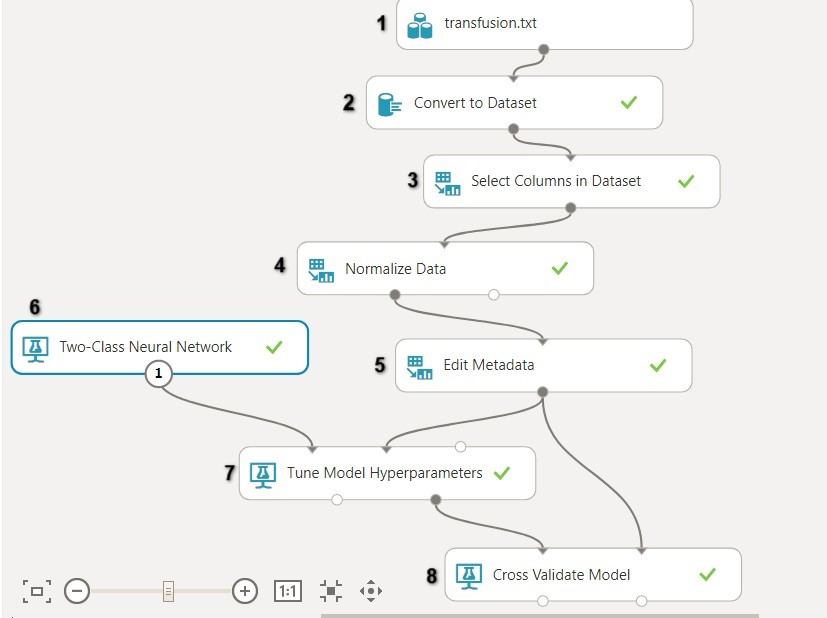In recent years machine learning (ML) tools have proved to be a very efficient in different subject's area. By current article we show how to apply ML classification methods to the blood donation system, the field we are particularly interested in DonorUA project (smart blood donor recruitment system). The main goal is to predict whether one will probably donate blood in a certain period of time (e.g. month) depending on some set of parameters (so-called “features”). We will show the full ML process, including building, training and testing the model.

In machine learning and statistics, classification is the problem of identifying to which of a set of categories (classes, sub-populations) a new observation belongs, on the basis of a training set of data containing observations (or instances) whose category membership is known. In our case, instances are donators, people who is registered to blood donation system and two classes – people who will donate and who wont (in the current month). Each instance is represented by a set of attributes (“features”) values, that we assume to influence belonging to either one of the defined categories.

To solve our classification problem, we need “training” dataset – dataset of donators about each of whom we know whether he/she donated blood in a certain month. The appropriate dataset is available at UCI Machine Learning Repository. It contains 748 instances, each instance represented by 5 feature values and labeled by 0 (did not donate in March 2007) or 1 (donated in March 2007).

Features are following:

• Recency – months since last donation
• Frequency – total number of donation
• Monetary – total blood donated in c.c.
• Time – months since first donation

We assume that our data is probably non-linearly separable, therefore, we need to choose ML model that allows to solve non-linear classification problems. One of the simplest of such models is feedforward neural network FFNN with one hidden layer (one hidden layer is usually sufficient). In case of linear separability FFNN is quite redundant, but still it should classify instances properly. Thus, pick of this model seems to be reasonable.

Firstly, we elaborate our problem step by step in Azure ML Studio. Afterwards, the same model is built via mxnet R package and results obtained in both cases are compared.

In order to develop a model, one need to create an experiment. We have already done that, so we just click on it to proceed (see picture below).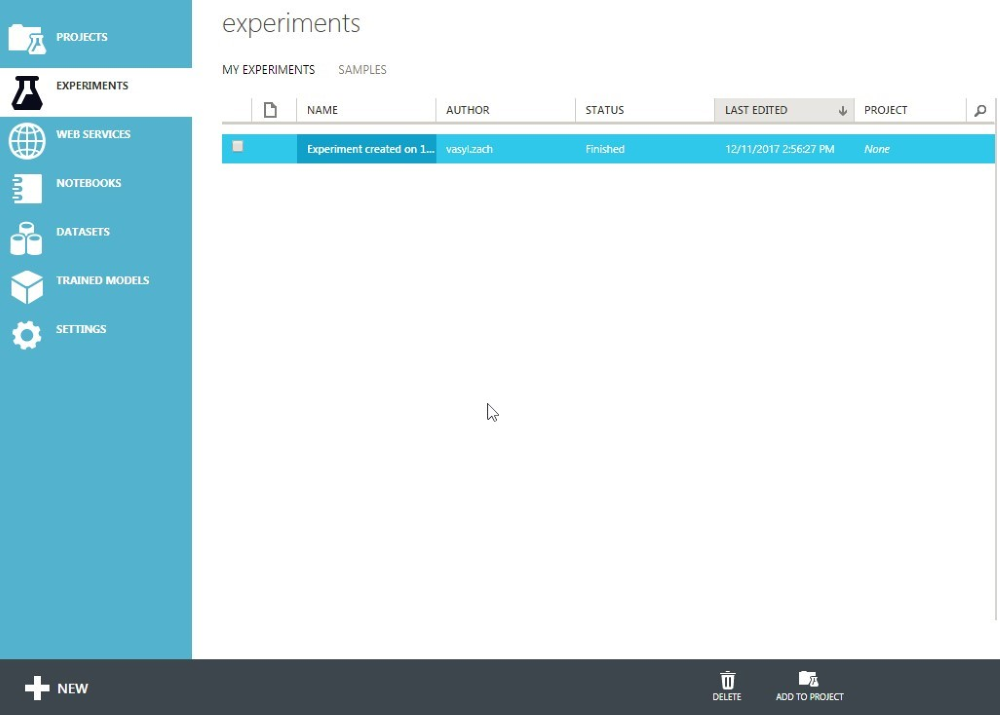Azure ML Studio is high-level environment designed for building ML models. It provides modules, allowing you to prepare and transform data, configure, train and evaluate ML models, and make predictions using saved models. You drag modules you want from the left panel onto canvas, connect them and then run the experiment. The scheme for our model you can see on the picture below.Now, let us gradually explain the workflow of the experiment:

#### Data preparation

1) Saved Datasets module. We add previously saved data into experiment - transfusion.csv.

2) Convert To Dataset module. We convert .csv to Azure ML Studio internal data format.

3) In Select Columns in Dataset module we can include/exclude dataset columns, thus vary model features.

4) Normalize Data module. Before training data can be plugged as input into neural network it has to be normalized. We do MinMax normalization $$x{i}^{j'} = \frac{x{i}^{j}-min{j}x{i}^{j}}{max{j}x{i}^{j}-min{j}x{i}^{j}}$$, thus after normalization procedure all feature values lie in (0,1) range.

5) In Edit Metadata module, we select which column is a label one (contains information about class of instance).

#### Configuring model (neural network)

6) Two-Class Neural Network module represents feed forward neural network algorithm with one hidden layer for binary classification problem.

Here we set our neural network to be fully connected (each input perceptron connected with each hidden, each hidden - to each output). Also, we define here the number of hidden nodes (number of neurons in hidden layer, we will vary this it from 2 to 8). Setting create trainer mode to Parameter Range and by selecting property use range builder for learning rate and number of iterations allows us to find the best model in terms of learning rate and number of iterations meanings automatically using the Tune Model Hyperparameters (see step 7).

Shuffle examples attribute ensures that instances are randomly reordered between iterations.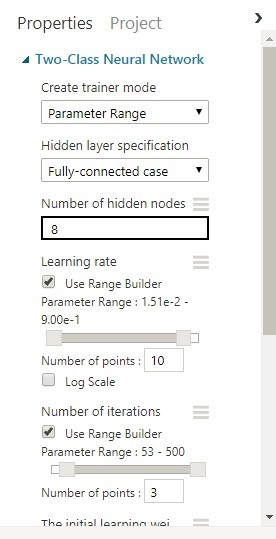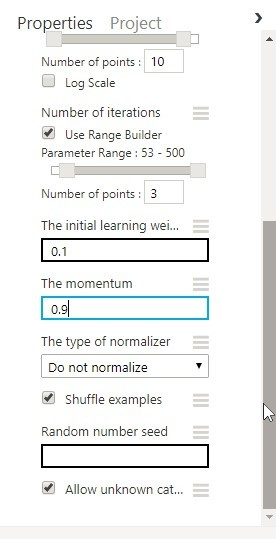#### Training the model

7) Tune Model Hyperparameters module automatically chooses the best model in terms of its hyperparameters (learning rate and number of iterations in our case). We assign parameter sweeping type to Random grid and “maximum runs on random grid” to 50. It means, that system randomly chooses 50 combinations of hyperparameters from the range defined on step 6, will train 50 corresponding models using training set and output the best one evaluated by chosen metric (accuracy, fraction of correct outputs).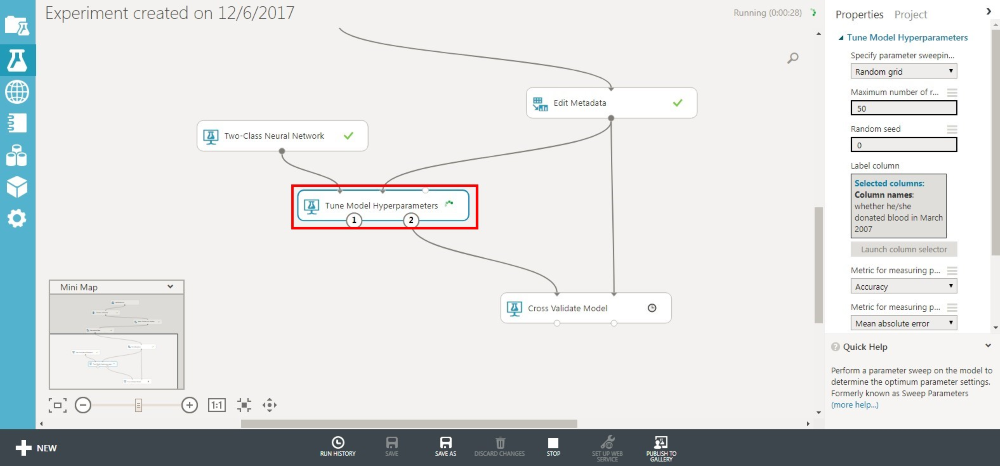#### Evaluating the model

8) Cross Validate Model module. In order to estimate generalization error we use cross-validation technique, in particular leave-p-out cross validation. Leave-p-out cross-validation (LpO CV) involves using p observations as the validation set and the remaining observations as the training set. This is repeated on all ways to cut the original sample on a validation set of p observations and a training set (https://en.wikipedia.org/wiki/Cross-validation_(statistics)). Cross Validate Model module provides us with tools to do that. We plug into it the best model from Tune Model Hyperparameters. Note, that the best model has already been trained, therefore in such a scenario, Cross Validate Model is used to test model (checks it predicting reliability) outputted by Tune Model Hyperparameters and generates evaluation metrics (accuracy, precision, recall, etc.).

### Results

In this section we present the results for different configurations of FFNN (different number of neurons in hidden layer in the case). There are empirical rules-of-thumb for determining the number of neurons in hidden layer. We list some of them (Introduction to Neural Networks for Java, Second Edition, Jeff Heaton):

• The number of hidden neurons should be between the size of the input layer and the size of output layer.
• The number of hidden neurons should be 2/3 the size of the input layer, plus the size of the output layer.
• The number of hidden neurons should be less than twice the size of the input layer.

So, we consider configurations with $$N{h} =$$ 2, 3, 4 and 8 hidden neurons. On pictures below metrics by fold and mean metrics are presented for each $$N{h}$$ value.

1) $$N_{h} = 2$$, learning_rate = 0.103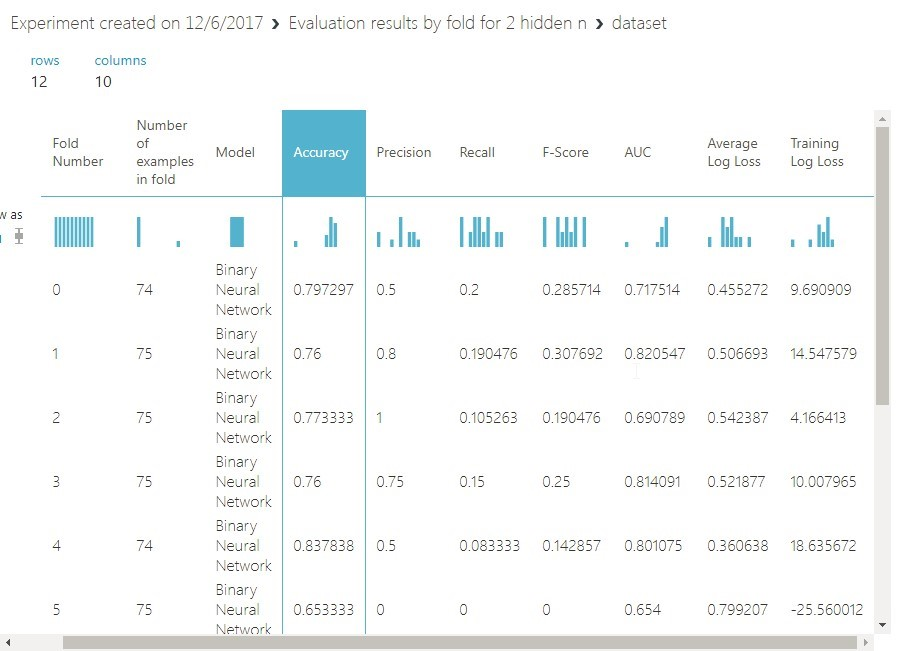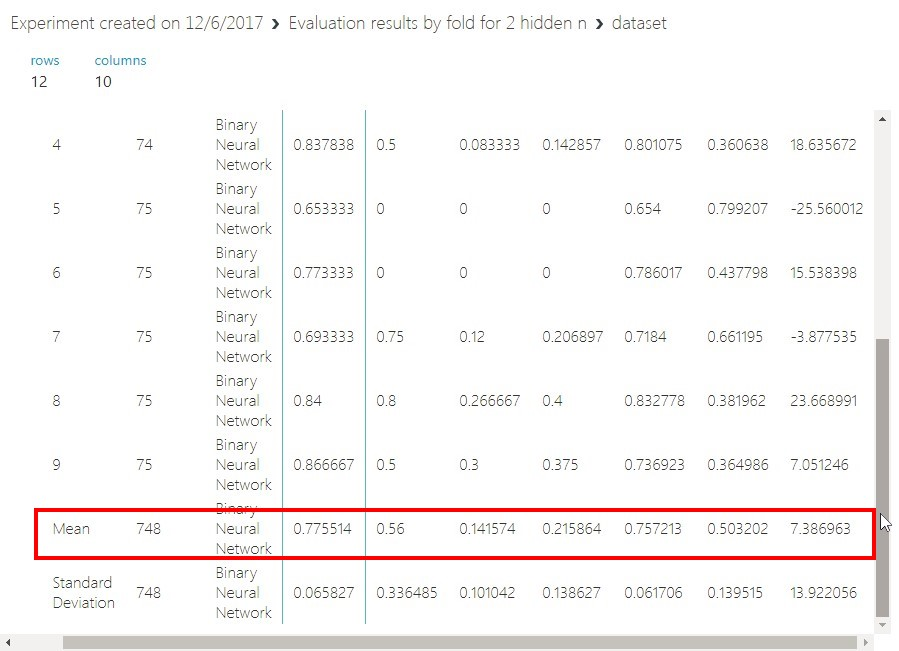2) $$N_{h} = 3$$, learning_rate = 0.103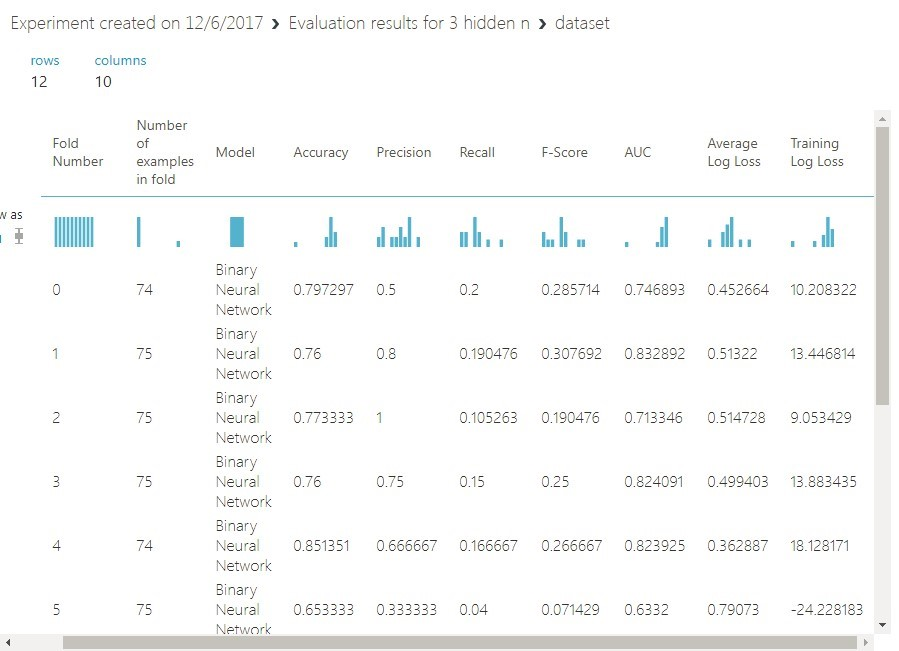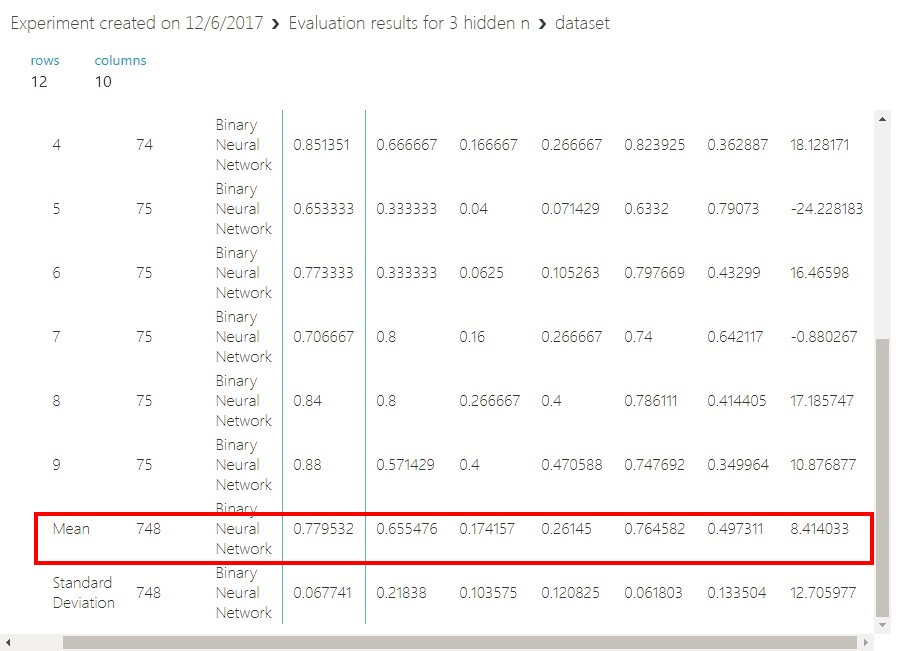3) $$N_{h} = 4$$, learning_rate = 0.192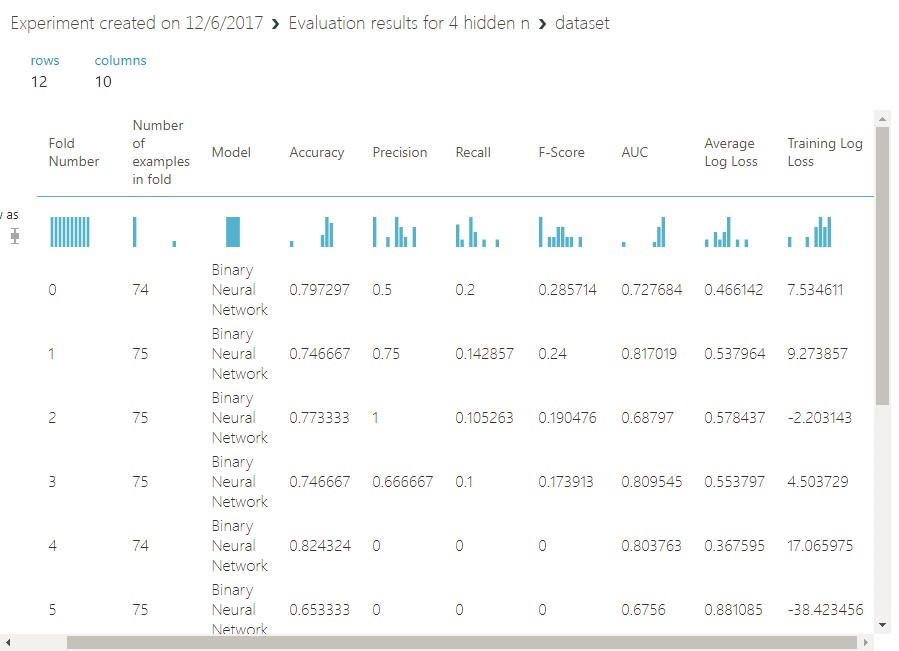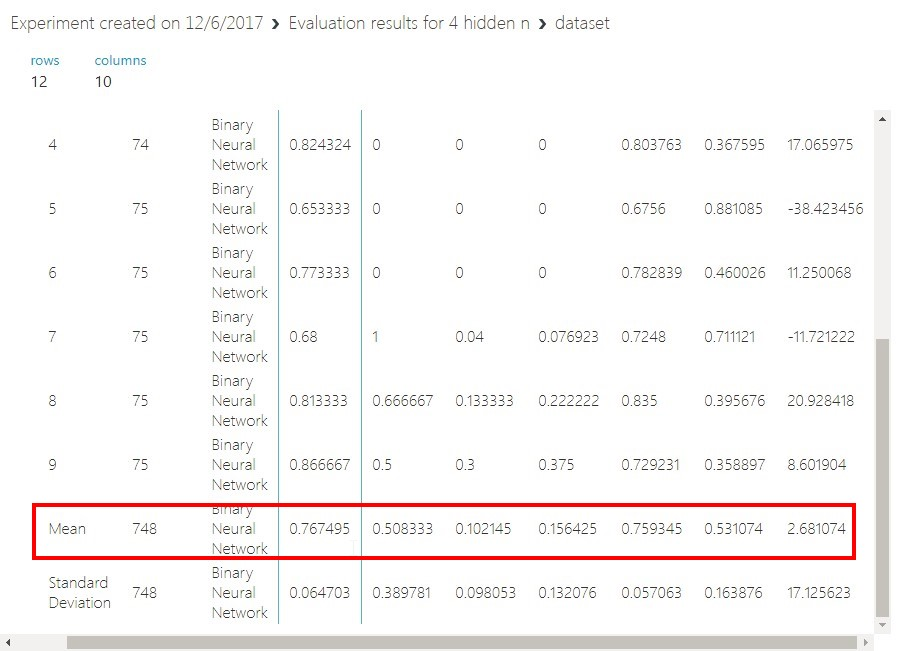4) $$N_{h} = 8$$, learning_rate = 0.104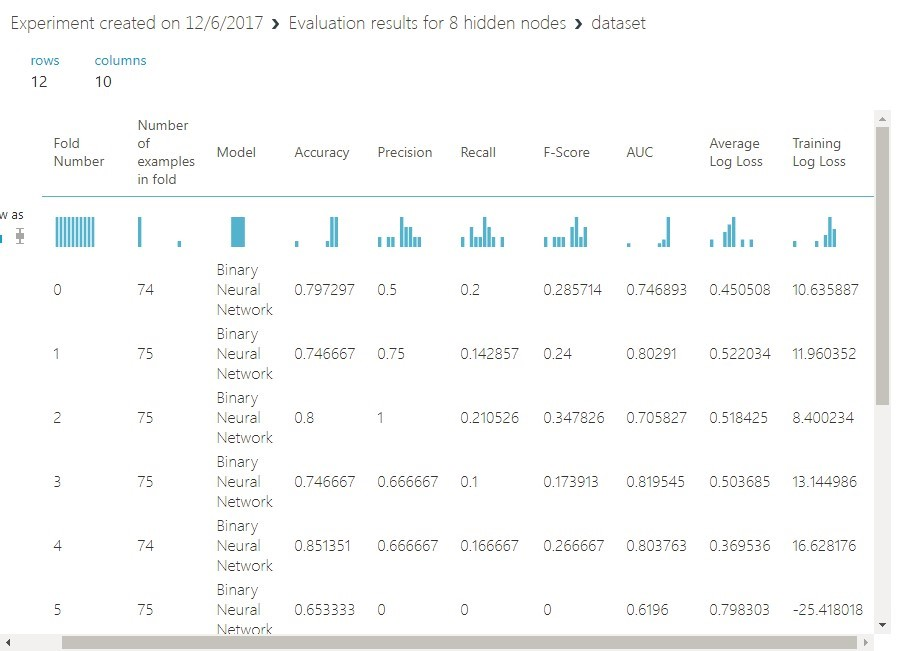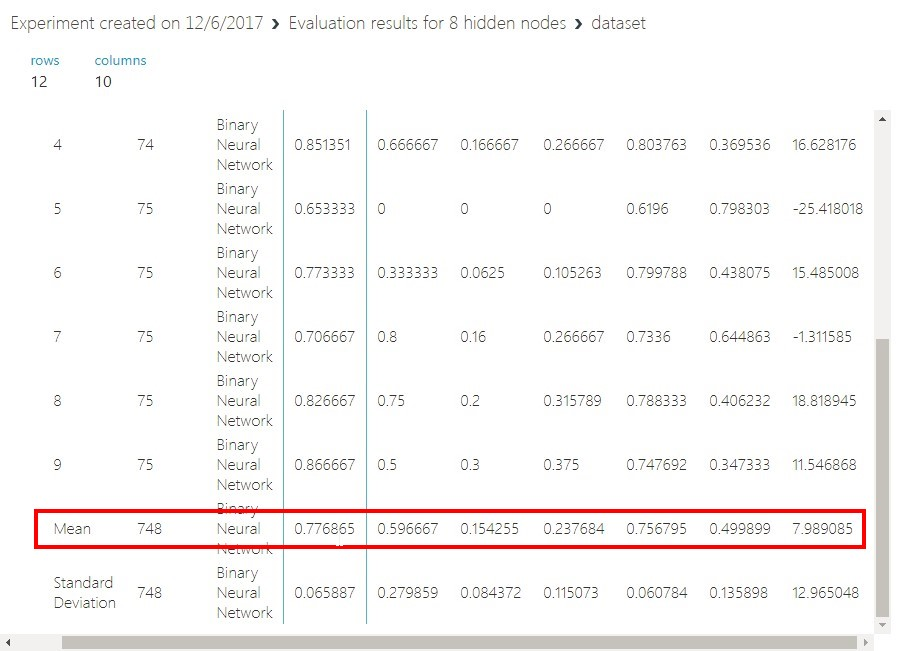No. hidden nodes Accuracy Precision Recall F-Score AUC Average Log Loss Training Log Loss
2 0.775514 0.56 0.141574 0.215864 0.757213 0.503202 7.386963
3 0.779532 0.655476 0.174157 0.26145 0.764582 0.497311 8.414033
4 0.767495 0.508333 0.102145 0.156425 0.759345 0.531074 2.681074
8 0.776865 0.596667 0.154255 0.237684 0.756795 0.499899 7.989085

Let’s analyze received results. First of all, it seems metrics that are approximately the same for for all network configurations. In our opinion model with 3 nodes (FFNN3) is the best one, due to the highest precision and recall values. Precision characterizes the accuracy of our model while predicting positive class instances, and recall – how complete were those predictions on positive class instances (see picture below).So, 66% of people who was predicted to donate blood by FFNN3 really donated it, while 73% of people who donated blood were not considered as such by FFNN3. To understand how good these results are lets look at scatter graphs of initial data. (15-18)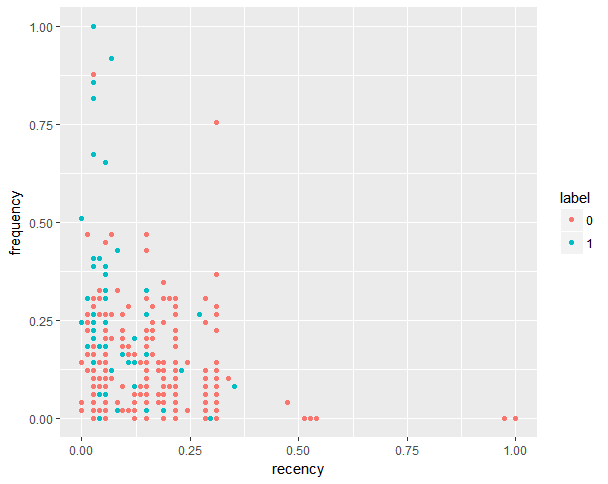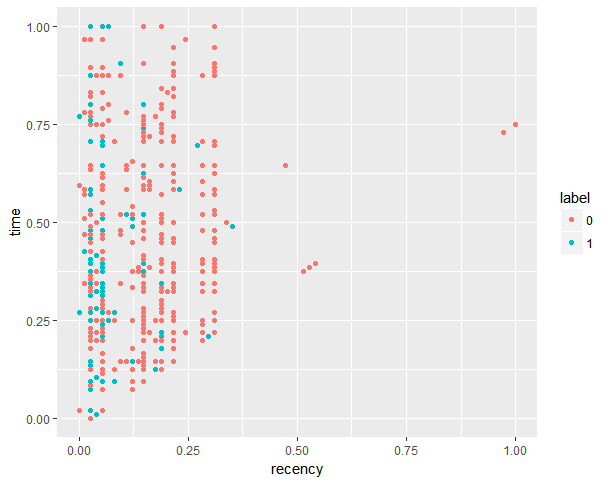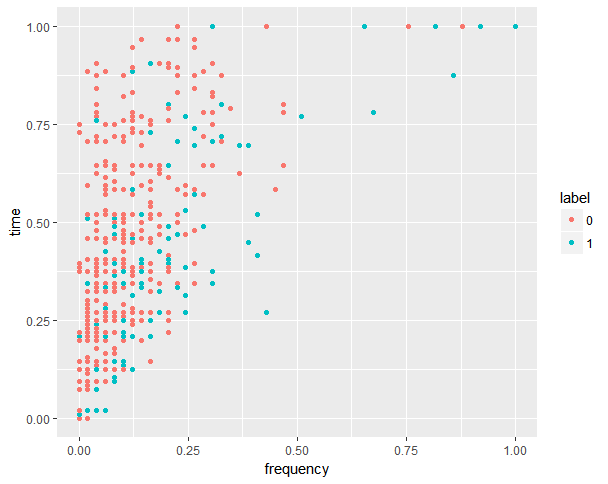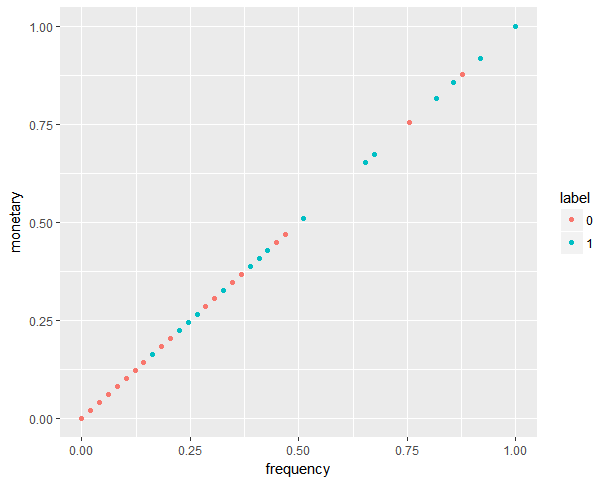As you can see normalized monetary values are exactly equal to frequency values for corresponding instances, therefore we can drop out recency-monetary and time-monetary graphs.

Considering graphs above, we conclude that classes domains are not well defined, they are overlapping each other. This can explain the tolerance of predicting results to model configuration. We suppose, that to estimate how good current models are, at least in terms of “precision” metrics, more data on people who did donate blood is needed.

To resolve “non-separability”, some non-linear transformations of feature space can be tried, as well as adding some new features in order to make domains of our classes distinct in enlarged spaces.

Finally, to approve received results, we build the same model in different environment, particularly in R using mxnet package.

To install mxnet on Windows use commands:

cran <- getOption("repos")
cran["dmlc"] <- "https://apache-mxnet.s3-accelerate.dualstack.amazonaws.com/R/CRAN/"
options(repos = cran)
install.packages("mxnet", dependencies = TRUE)


For other platforms see https://mxnet.incubator.apache.org/install/index.html.

#### Data preparation

#importing data from local storage and renaming columns
names(data) = c("recency", "frequency", "monetary", "time", "classes")

#dividing data into training and test datasets (0.8 and 0.2 of initial data)
indexes = sample(1:nrow(data), size=0.2*nrow(data))
test = data[indexes,]
train = data[-indexes,]

train.x = train[, 1:4]
train.y = train[, 5]

test.x = data.matrix(test[, 1:4])
test.y = test[, 5]


In R we used another approach to model train and evaluation called Simple Cross Validation. We randomly split our data to train set (80% of data) and test set (20%). The model is trained on train set and then is evaluated on test set.

#### Configuring model

#input layer
data <- mx.symbol.Variable("data")
#first hidden layer
fc1 <- mx.symbol.FullyConnected(data, num_hidden = 3, name = "fc1")
relu1 <- mx.symbol.Activation(fc1, act_type = "relu", name = "relu1")
#output layer
fc2 <- mx.symbol.FullyConnected(relu1, num_hidden = 2, name = "fc2")
sfo <- mx.symbol.SoftmaxOutput(fc2, name = "lro")


In the snippet above, using mxnet library we defined configuration for FFNN with one hidden layer that contains 3 neurons, relu is chosen to be activation function (https://en.wikipedia.org/wiki/Activation_function). Output activation function set to softmax (this function will output the vector of probabilities for instance to belong to each class). Our choice of output function also implies that loss function is cross-entropy loss function (https://en.wikipedia.org/wiki/Loss_function).

#### Training the model

model <- mx.model.FeedForward.create(
sfo, X = data.matrix(train.x), y = train.y, num.round = 351, array.batch.size = 50,
learning.rate= 0.103, momentum = 0.9, array.layout="rowmajor",
eval.metric = mx.metric.accuracy,
)


In the snippet above we set values for hyperparameters (for each considered number of hidden neurons we take values for learning rate (learning.rate) and number of iterations(num.round) that were the best for corresponding FFNN configuration in Azure ML Studio experiment)

#### Evaluating the model

In order to evaluate the model, we run prediction operation on the test set, and after that compute evaluation metrics. Getting prediction results made by trained FFNN on test set is made by simple command in mxnet library:

preds = predict(model, test.x)
`

## other posts

Sunday, November 10, 2019 12:38 PM

### Blazor: new way to build Web UI

New way to build UI with C# & .NET

Monday, May 20, 2019 9:31 PM

### Flutter: Yes or No?

Flutter is a new way to build apps for mobile, desktop and web platforms. A first look from Xamarin developer.

Thursday, May 2, 2019 6:23 PM

### DevRain joins the Microsoft AI Inner Circle Partner Program

The AI Inner Circle Partner program is designed for partners who provide custom services or enhanced AI product solutions utilizing Microsoft AI technologies.